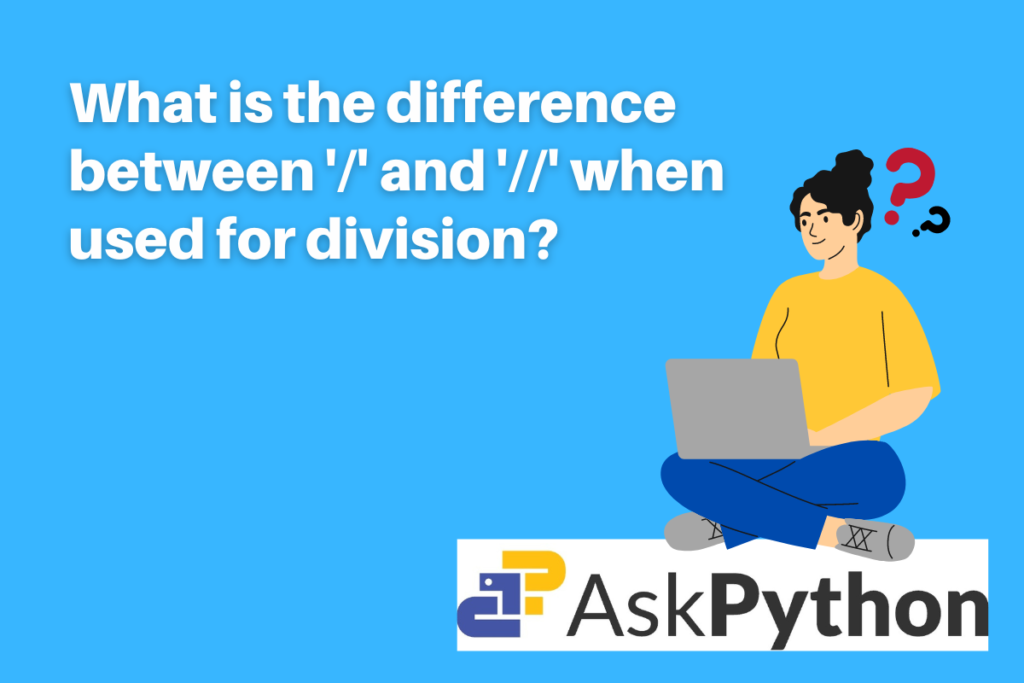# Difference between ‘/’ and ‘//’ in Python divisionLet’s try to understand the difference between the ‘/’ and the ‘//’ operators used for division in the Python. Before getting there first, let’s take a quick look into what is the need for a division operator.

## What is a division operator in Python?

Python programming language provides various arithmetic operators. Arithmetic operators are operators that help in performing arithmetic operations between two operands (numbers). One such arithmetic operation is the division operation.

It provides the quotient of the two input operands as the output. The number before the operator is considered the dividend while the number that comes after the operator is considered the divisor.

## Difference between the ‘/’ and the ‘//’ division operators in Python

There are two ways to carry out division in Python with a slight difference in the output. Let’s look at both of them in detail.

### 1. Performing division using the ‘/’ operator

This method of division is considered as the ‘classic division’. The ‘/’ single slash carries out the float division. The output of this operator is always a quotient with a float datatype. The output remains float even if the input numbers are integer values. Even the sign of the input operands doesn’t matter about the output.

Examples

```#integer input
x1 = 15
y1 = 6
print(x1/y1)
```

Output

```#float input
x2 = 15.0
y2 = 3.0
print(x2/y2)
```

Output

Note that in both of the above cases, the output is the same even when the input datatypes are different.

```#negative input
x3 = -15
y3 = 2
print(x3/y3)
```

### 2. Performing division using the ‘//’ operator

This method of division is considered the ‘true division’. The ‘//’ double slash carries out integer division which is also known as floor division. The output of this operator will be the quotient rounded off to the closest whole number. For example, 15 divided by 6 is actually 2.5 but it gets rounded off to 2.

The data type of the output, in this case, is an integer for integer input whereas floating for float input. In the case of the negative input number, the output is rounded off to the larger side. For example, -15 divided by 6 is -2.5, since the quotient has a negative sign assigned to it, the output of floor division will get rounded off to -3 (remember not -2.)

The working of this operator is the same as that of math.floor function

Examples

```#integer input
x1 = 15
y1 = 6
print(x1//y1)
```

Output

```#float input
x2 = 15.0
y2 = 6
print(x2//y2)
```

Output

```#negative input
x2 = 15.0
y2 = 6
print(x2//y2)
```

Output

Note that when a negative value is passed as input, in floor division the greater integer number is in the output.

## What is the need for two operators for division?

• The classic division operator is considered to be a single slash in python. Though it carries out proper division, the problem is it returns floating output even for integer and long input values and this might cause an error in some situations. Many functions for example range() expects integer as the data type of the output and passing integer values with the ‘/’ operator will be syntactically correct but will surely raise TypeError as the output will not have an acceptable data type.
• On the other hand the double slash operator ‘//’ always rounds off the quotient of the input value. This might cause mathematical errors in a situation where precise values and no approximation is expected. A very convenient alternative for this is to use the round() function or the floor() function and pass the classic division as it will yield the same results.

Note the above discussed differences are observed in Python 3.x series and not in the previous versions. in Python 2.x series ‘/’ is neither floor division nor true division. When both arguments are int, ‘/’ is floor division; however, if either argument is a float, it is true division.

## Summary

In conclusion, to solve the ambiguity problems there are two methods in Python to carry out division operations. The big question is which operator to prefer. It completely depends on the situation and the type of output expected.

To view more such detailed and easy-to-understand articles on Python programming language click here!

## Reference

https://peps.python.org/pep-0238/